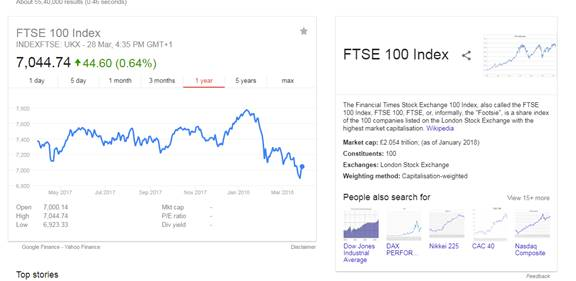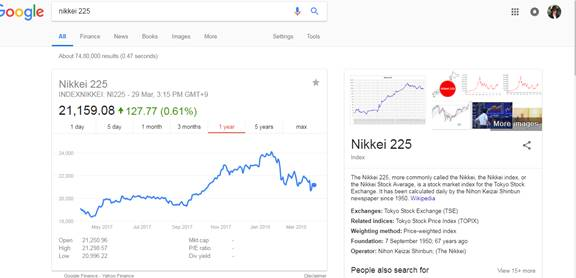Chapter 17, Problem 4DQ### Fundamentals of Financial Manageme...

9th Edition
Eugene F. Brigham + 1 other
ISBN: 9781305635937

#### Solutions

Chapter
Section### Fundamentals of Financial Manageme...

9th Edition
Eugene F. Brigham + 1 other
ISBN: 9781305635937
Textbook Problem
1 views

# Some of the websites provide information on the international indexes. a. How has the FTSE 100 (a U.K. stock, index) performed over the past year? Calculate its return (in local currency) over the past year. (Note: Index value is shown in local currency.) From your answer in question 3a, during this past year would a U.S. investor have earned more or less of a return calculated in dollars? Calculate the U.S. investor’s approximate dollar return. (Assume that the U.S. investor had originally invested $1,000 in a FTSE 100 index fund at the beginning of the year but wants to liquidate the investment at the end of the year. Ignore any transactions costs.) b. How has the Nikkei 225 (a Japanese stock index) performed over the past year? Calculate its return (in local currency) over the past year. (Note: Index value is shown in local currency.) From your answer in question 3b, during this past year would a us. investor have earned more or less of a return calculated in dollars? Calculate the U.S. investor’s approximate dollar return. (Assume that the U.S. investor had originally invested$1,000 in a N225 index fund at the beginning of the year but wants to liquidate the investment at the end of the year. Ignore any transactions costs.)a.

Summary Introduction

To determine: Performance and return on stock.

Introduction:

Stock Market:

Stock market is a secondary market where all the companies are listed and the shares of the listed company is traded.

Explanation

F-100 performance is not good over the past year.

Given,

One year ago F-100 stock price was $7,373. Today F-100 stock price is$7,044.

Formula to calculate the returns,

Return=OldpriceNewprice

Substitute $7,373 for the old price, and$7,044 for the new price.

Return=$7,373$7,044=$329 • It can be said that performance of the dollar one year ago was better than now so is can be said the US investor would get more return on the investment. • As the US investor getting more return from the investment so investor will want to invest. Formula to calculate the Investor’s approximate dollar return, Investorreturen=Investedamount×Percentageofdecreaseinthereturn Substitute,$1,000 for the invested amount and 95

b.

Summary Introduction

To determine: Approximate dollar return.

### Still sussing out bartleby?

Check out a sample textbook solution.

See a sample solution

#### The Solution to Your Study Problems

Bartleby provides explanations to thousands of textbook problems written by our experts, many with advanced degrees!

Get Started